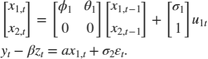# Implicitly Create State-Space Model Containing Regression Component

This example shows how to implicitly create a state-space model that contains a regression component in the observation equation. The state model is an ARMA(1,1).

Write a function that specifies how the parameters in params map to the state-space model matrices, the initial state values, and the type of state. Specify the regression component by deflating the observations within the function. Symbolically, the model is:% Copyright 2015 The MathWorks, Inc. function [A,B,C,D,Mean0,Cov0,StateType,DeflateY] = regressionParamMap(params,y,z) % State-space model with a regression component parameter mapping function % example. This function maps the vector params to the state-space matrices % (A, B, C, and D), the initial state value and the initial state variance % (Mean0 and Cov0), and the type of state (StateType). The state model is % an ARMA(1,1). varu1 = exp(params(3)); % Positive variance constraint vare1 = exp(params(4)); A = [params(1) params(2); 0 0]; B = [sqrt(varu1); 1]; C = [1 0]; D = sqrt(vare1); Mean0 = [0.5 0.5]; Cov0 = eye(2); StateType = [0 0]; DeflateY = y - params(5)*z; end 

Save this code as a file named regressionParamMap on your MATLAB® path.

Create the state-space model by passing the function regressionParamMap as a function handle to ssm.

Mdl = ssm(@(params)regressionParamMap(params,y,z)); 

ssm implicitly creates the state-space model. Usually, you cannot verify implicitly defined state-space models.

Before creating the model, ensure that the data y and z exist in your workspace.